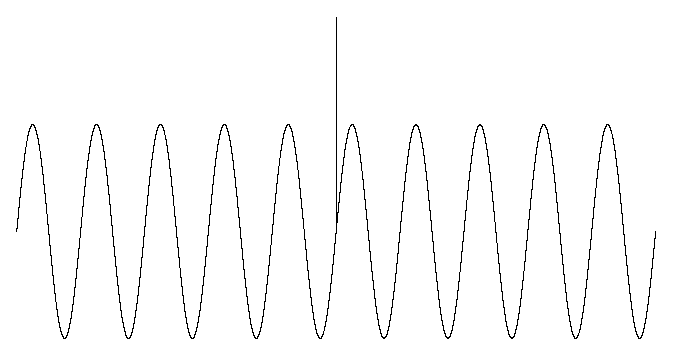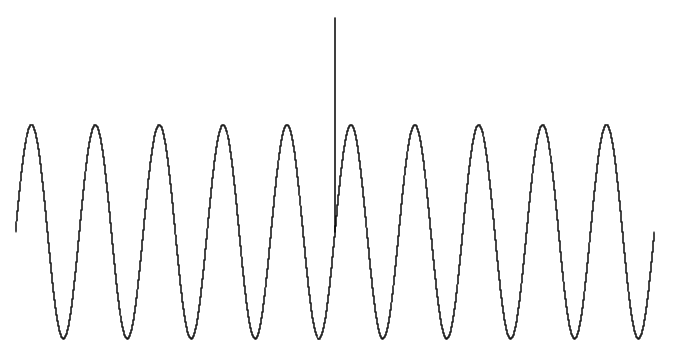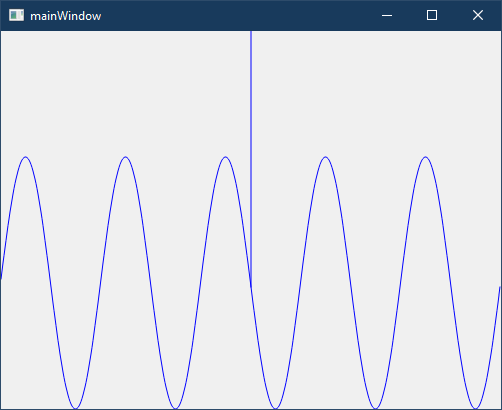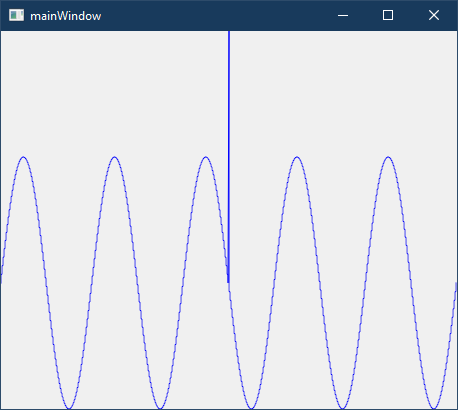# How to improve anti-aliasing?

• Hi! I am trying to make a line plot in `QWidget`. To obtain the line plot I map the X and Y points of my data point to its correct position in `QWidget` and then draw a line using `QPainter`'s `drawLine()` function connecting previous point to the current point. The data points are huge (in tens or hundreds of thousands). What I get is the following:

Aliased:Anti-alised:In both of the above examples, the pen width is 1 and 100,000 data points are plotted in a sine wave with frequency 10 and amplitude 1. There is one data point at the center which is of value 2 which stands out, this is because I need to accommodate spikes in my plot. Since I need to accommodate spikes, I cannot use any kind of algorithm based smoothing (such as using Bezier Curves). This leaves me with options such as anti-alising and painting tricks, but as you can see above anti-alising doesn't produce a good result. I have tried the following as well:

• Using `double` precision instead of `integer` precision for mapping the X and Y data points to it's correct position on `QWidget`. This significantly increases the calculation time but the final plot looks the same as if I would have done the calculation with integer precision.
• Painting my line plot on a `QImage` 4 times the size of the plot area, then applying anti-alising to it and then shrink it to correct size. This also increases calculation time significantly but the final plot again looks the same as above.

I don't think using OpenGL Paint Engine is a good idea as explained in this QOpenGLPaintDevice Class document. Is there any way I can improve the rendering of my line plots?

• @CJha Found a solution. This works quite fast, 100k data points plotted in around 15 - 20 ms.

``````void mainWindow::paintEvent(QPaintEvent *event)
{
/* Constructing Line Plot */
double numOfPoints{100000}; // Data Length is 100 Thousand
QVector<double> data; // Data Vector of 100 Thousand
for(int ii = 0; ii < numOfPoints; ++ii)
{
// Sine Wave: Amplitude = 1; Frequency = 10
data << (1 * sin (2 * M_PI * 5 * ii/numOfPoints));
}
int midPoint = round(numOfPoints/2);
data[midPoint] = 2; // Adding a single point of amplitude 2 at 50 thousandth data point
double dataMin{-1}; // Minimum amplitude of data
double dataMax{2}; // Maximum amplitude of data

/* Setting up Painter */
QPainter p(this);
p.setPen(Qt::blue);
p.setRenderHint(QPainter::Antialiasing);
QPolygonF polyline; // To draw lines to connect two points with different x-coordinates
QPainterPath path; // To draw abstract lines with same x-coordinate but different y-coordinates

/* NOTE: QPainter path is used because it has a moveTo functionality i.e. path's coordinates can be changed without creating a line from previous coordinate to new one. Same is not true for QPolygonF and so it is used to draw the continuous line */

/************************************************
***** Mapping Data to rect() of mainWindow *****
************************************************/
QVector<int> py; // Point Y
QVector<int> sy; // Serial Y
QVector<int> sySize; // Sizes of sy
QVector<double> val; // Y Value for different groups of data
int x_cur{0}; // x-value for current data set
int x_prv{0}; // x-value for previous data set
int y{0};
int majy{0}; // Major Y i.e. the value which QPolygonF will follow
double miny{0}; // Minimum Y: Start Point of QPainterPath
double maxy{0}; // Minimum Y: End Point of QPainterPath
int idy{0}; // ID to identity Value of Major Y

/* This only works for data size larger than width of screen. For data size smaller than screen a much simpler approach is used but is not shown here */
if(data.size() > width())
{
path.clear();
for(int ii = 0; ii < (width() + 1); ++ii)
{
py.clear();
x_cur = round(ii * (numOfPoints/width()));
if(ii != 0)
{
for(int jj = x_prv; jj < x_cur; ++jj)
{
y = round(height() * (dataMin + (dataMax - dataMin) - data[jj]) / (dataMax - dataMin));
if(!py.contains(y)) { py << y; }
}
if(py.size() == 1) { polyline << QPointF((ii - 1.0), py); }
else
{
std::sort(py.begin(), py.end());
sy.clear();
sySize.clear();
val.clear();
majy = 0;
miny = 0.0;
maxy = 0.0;
idy = 0;
for(int jj = 0; jj < py.size(); ++jj)
{
if(jj == 0) { sy << py[jj]; }
else
{
if(py[jj] == (py[jj - 1] + 1)) { sy << py[jj]; }
else
{
double newVal{0.0};
for(int kk = 0; kk < sy.size(); ++kk)
{
newVal = newVal + sy[kk];
}
val.append(newVal / sy.size());
sySize << sy.size();
sy.clear();
sy << py[jj];
}
}
}
double newVal{0.0};
for(int kk = 0; kk < sy.size(); ++kk)
{
newVal = newVal + sy[kk];
}
val.append(newVal / sy.size());
sySize << sy.size();
if (val.size() == 1) { polyline << QPointF((ii - 1.0), val); }
else
{
for(int kk = 0; kk < sySize.size(); ++kk)
{
if(sySize[kk] > majy)
{
majy = sySize[kk];
idy = kk;
}
}
auto result = std::minmax_element(val.begin(), val.end());
miny = *result.first;
maxy = *result.second;
polyline << QPointF((ii - 1.0), val[idy]);
path.moveTo(QPointF((ii - 1.0), miny));
path.lineTo(QPointF((ii - 1.0), maxy));
}
}
}
x_prv = x_cur;
}
}
p.drawPolyline(polyline);
p.drawPath(path);
}
``````

At the end this is what I get:• Hi
I could not reproduce it as such.Maybe your pixels are a bit off but you said you used doubles and i assume you then
also used the QPointF version ?

``````void MainWindow::paintEvent(QPaintEvent *event)
{
QPainter p(this);
p.setPen( Qt::blue );
p.setRenderHint( QPainter::Antialiasing );
QPolygonF polyline;
int h_div_2 = height() / 2;
for( int x = 0; x < width(); x++)
{
float y = h_div_2 + sin( x * 6.28f / width() ) * h_div_2;
polyline << QPointF( x, y );
}
p.drawPolyline( polyline );
}
``````

• @mrjj Thanks for the reply. You are constructing the data based on the size of your `MainWindow` i.e. you are using the `width()` of the `MainWindow` in your calculation of sine wave, this will always produce a smooth curve because it will calculate the exact y-coordinate for each x-coordinate of your `MainWindow`. I have to plot the recorded data with high sampling frequency i.e. the data that I am trying to plot is independent of the `width()` and `height()` of my `mainWindow` and so I have to map it to my `mainWindow`:

``````void mainWindow::paintEvent(QPaintEvent *event)
{
/* Constructing Demo Line Plot */
int numOfPoints{100000}; // Data Length is 100 Thousand
QVector<double> data; // Data Vector of 100 Thousand
for(int ii = 0; ii < numOfPoints; ++ii)
{
data << (1 * sin (2 * M_PI * 5 * ii/numOfPoints)); // Sine Wave: Amplitude = 1; Frequency = 5
}
data = 2; // Adding a single point of amplitude 2 at 50 thousandth data point
double dataMin{-1.0}; // Minimum amplitude of data
double dataMax{2.0}; // Maximum amplitude of data

/* Setting up Painter */
QPainter p(this);
p.setPen(Qt::blue);
p.setRenderHint(QPainter::Antialiasing);
QPolygonF polyline;

/* Mapping data to rect() of mainWindow */
for(int ii = 0; ii < numOfPoints; ++ii)
{
double px = width() * ii / numOfPoints;
double py = height() * (dataMin + (dataMax - dataMin) - data[ii]) / (dataMax - dataMin);
polyline << QPointF(px, py);
}
p.drawPolyline(polyline);
}
``````

This produces the following result:The lines are not smooth, and I don't know how to fix it.

• @CJha Found a solution. This works quite fast, 100k data points plotted in around 15 - 20 ms.

``````void mainWindow::paintEvent(QPaintEvent *event)
{
/* Constructing Line Plot */
double numOfPoints{100000}; // Data Length is 100 Thousand
QVector<double> data; // Data Vector of 100 Thousand
for(int ii = 0; ii < numOfPoints; ++ii)
{
// Sine Wave: Amplitude = 1; Frequency = 10
data << (1 * sin (2 * M_PI * 5 * ii/numOfPoints));
}
int midPoint = round(numOfPoints/2);
data[midPoint] = 2; // Adding a single point of amplitude 2 at 50 thousandth data point
double dataMin{-1}; // Minimum amplitude of data
double dataMax{2}; // Maximum amplitude of data

/* Setting up Painter */
QPainter p(this);
p.setPen(Qt::blue);
p.setRenderHint(QPainter::Antialiasing);
QPolygonF polyline; // To draw lines to connect two points with different x-coordinates
QPainterPath path; // To draw abstract lines with same x-coordinate but different y-coordinates

/* NOTE: QPainter path is used because it has a moveTo functionality i.e. path's coordinates can be changed without creating a line from previous coordinate to new one. Same is not true for QPolygonF and so it is used to draw the continuous line */

/************************************************
***** Mapping Data to rect() of mainWindow *****
************************************************/
QVector<int> py; // Point Y
QVector<int> sy; // Serial Y
QVector<int> sySize; // Sizes of sy
QVector<double> val; // Y Value for different groups of data
int x_cur{0}; // x-value for current data set
int x_prv{0}; // x-value for previous data set
int y{0};
int majy{0}; // Major Y i.e. the value which QPolygonF will follow
double miny{0}; // Minimum Y: Start Point of QPainterPath
double maxy{0}; // Minimum Y: End Point of QPainterPath
int idy{0}; // ID to identity Value of Major Y

/* This only works for data size larger than width of screen. For data size smaller than screen a much simpler approach is used but is not shown here */
if(data.size() > width())
{
path.clear();
for(int ii = 0; ii < (width() + 1); ++ii)
{
py.clear();
x_cur = round(ii * (numOfPoints/width()));
if(ii != 0)
{
for(int jj = x_prv; jj < x_cur; ++jj)
{
y = round(height() * (dataMin + (dataMax - dataMin) - data[jj]) / (dataMax - dataMin));
if(!py.contains(y)) { py << y; }
}
if(py.size() == 1) { polyline << QPointF((ii - 1.0), py); }
else
{
std::sort(py.begin(), py.end());
sy.clear();
sySize.clear();
val.clear();
majy = 0;
miny = 0.0;
maxy = 0.0;
idy = 0;
for(int jj = 0; jj < py.size(); ++jj)
{
if(jj == 0) { sy << py[jj]; }
else
{
if(py[jj] == (py[jj - 1] + 1)) { sy << py[jj]; }
else
{
double newVal{0.0};
for(int kk = 0; kk < sy.size(); ++kk)
{
newVal = newVal + sy[kk];
}
val.append(newVal / sy.size());
sySize << sy.size();
sy.clear();
sy << py[jj];
}
}
}
double newVal{0.0};
for(int kk = 0; kk < sy.size(); ++kk)
{
newVal = newVal + sy[kk];
}
val.append(newVal / sy.size());
sySize << sy.size();
if (val.size() == 1) { polyline << QPointF((ii - 1.0), val); }
else
{
for(int kk = 0; kk < sySize.size(); ++kk)
{
if(sySize[kk] > majy)
{
majy = sySize[kk];
idy = kk;
}
}
auto result = std::minmax_element(val.begin(), val.end());
miny = *result.first;
maxy = *result.second;
polyline << QPointF((ii - 1.0), val[idy]);
path.moveTo(QPointF((ii - 1.0), miny));
path.lineTo(QPointF((ii - 1.0), maxy));
}
}
}
x_prv = x_cur;
}
}
p.drawPolyline(polyline);
p.drawPath(path);
}
``````

At the end this is what I get: